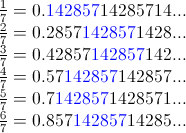Main

## Numbers worth knowing: 142857

Filed in Encyclopedia ,IdeasSubscribe to Decision Science News by Email (one email per week, easy unsubscribe)

ONE CRAZY NUMBER

We at DSN thought it would be worth memorizing some reciprocals because we have a system for remembering numbers and because it might come in handy. So, we started writing out 1/x

1/2 = .5
1/3 = .3
1/4 = .25
1/5 = .2
1/6 = .16
1/7 = .142857
1/8 = .125
1/9 = .1
1/10 = .1

Everything seems plain, predictable, ordinary, but what’s that going on at 1/7? .142857 repeating. That’s weird. Everywhere else it’s the first or second digit right of the decimal that repeats, but then at 1/7 you get 6 unique numbers that repeat as a weird group.

Are the multiples of this number weird? They’re even weirder.

1/7 = .142857
2/7 = .285714
3/7 = .428571
4/7 = .571428
5/7 = .714285
6/7 = .857142
See what’s going on there? They’re all just rotations of the same digits, 142857. Just pop digits off the left and stick them on the right to get any of the above.

Or as this blog shows it:If we keep going, we run across
22/7 = 3.142857, which is of course, very nearly (within .0013 of) pi. Weird.

100/7 = 14.2857142857, which is handy b/c it comes up a lot.

Now, we were hooked. A bit of search engine magic showed us that 142857 is kind of famous. It has its own Wikipedia page.

And it has other kooky propreties:
142+857=999 and
.142+.857 = 1

What’s more, 142857 is a Harshad number, which means it is divisible by the sum of its digits:
142857/(1+4+2+8+5+7) = 5291

What’s surely not the last oddity, if you write the numbers 1 … 9 around a circle, put a triangle connecting 3, 6, and 9 and then connect 1, 4, 2, 8, 5 and 7, you get the New-Agey enneagram pictured above.

Photo credit: Wikipedia.

1. Anonymous says:

And 142857 x 7 = 999999

November 11, 2016 @ 6:27 pm

2. Wiwin Wijaya says:

( 10^6 -1 ) / 7 = 142857

February 15, 2018 @ 6:11 am

3. Universal Ego says:

1000000000/7= 142857142.857
This will continue on as you ad more zeros

June 5, 2019 @ 8:29 pm

### Write Comment

XHTML: <a href="" title=""> <abbr title=""> <acronym title=""> <b> <blockquote cite=""> <cite> <code> <del datetime=""> <em> <i> <q cite=""> <s> <strike> <strong>